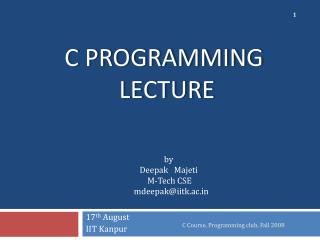# C Programming Lecture - PowerPoint PPT PresentationDownload PresentationC Programming Lecture

C Programming LectureDownload Presentation## C Programming Lecture

- - - - - - - - - - - - - - - - - - - - - - - - - - - E N D - - - - - - - - - - - - - - - - - - - - - - - - - - -
##### Presentation Transcript

1. C Course, Programming club, Fall 2008 C Programming Lecture by Deepak Majeti M-Tech CSE mdeepak@iitk.ac.in 17th August IIT Kanpur

2. Recap • C is a high-level language. • Writing a C code. {editors like gedit, vi} • Compiling a C code. {gcc –c test.c –o test} • Executing the object code. {./test} C Course, Programming club, Fall 2008

3. Some more basics • Keywords • char, static, if , while, return ..................... Total= about 32 • Data Types • int , char, float ...………..….. Some more later • Arithmetic Operators • + (Plus), - (Minus), * (Multiplication), /(Division) ……….………. Some more later C Course, Programming club, Fall 2008

4. My first C program! #include <stdio.h> // program prints hello world intmain() { printf ("Hello world!"); return0; } Output: Hello world! C Course, Programming club, Fall 2008

5. Example 1 #include <stdio.h> // program prints a number of type int intmain() { int number = 4; printf (“Number is %d”, number); return0; } Output: Number is 4 C Course, Programming club, Fall 2008

6. Example 2 #include <stdio.h> // program reads and prints the same thing intmain() { int number ; printf (“ Enter a Number: ”); scanf (“%d”, &number); printf (“Number is %d\n”, number); return0; } Output : Enter a number: 4 Number is 4 C Course, Programming club, Fall 2008

7. more and more #include <stdio.h> int main() { /* this program adds two numbers */ int a = 4; //first number int b = 5; //second number int answer = 0; //result answer = a + b; } C Course, Programming club, Fall 2008

8. Note Errors Compilation Compiler generally gives the line number at which the error is present. Run time C programs are sequential making the debugging easier. C Course, Programming club, Fall 2008

9. Some more Data Types • Primary : int, float, char • int (signed/unsigned)(2,4Bytes): used to store integers. • char (signed/unsigned)(1Byte): used to store characters • float, double(4,8Bytes): used to store a decimal number. • User Defined: • typedef: used to rename a data type • typedef int integer; can use integer to declare an int. • enum, struct, union C Course, Programming club, Fall 2008

10. Some more Arithmetic Operators • Prefix Increment : ++a • example: • int a=5; • b=++a; // value of b=6; a=6; • Postfix Increment: a++ • example • int a=5; • b=a++; //value of b=5; a=6; C Course, Programming club, Fall 2008

11. Contd… • Modulus (remainder): % • example: • 12%5 = 2; • Assignment by addition: += • example: • int a=4; • a+=1; //(means a=a+1) value of a becomes 5 Can use -, /, *, % also C Course, Programming club, Fall 2008

12. Contd… • Comparision Operators: <, > , <=, >= , !=, ==, !, &&, || . • example: • int a=4, b=5; • a<b returns a true(non zero number) value. • Bitwise Operators: <<, >>, ~, &, | ,^ . • example • int a=8; • a= a>>1; // value of a becomes 4 C Course, Programming club, Fall 2008

13. Operator Precedence • Meaning of a + b * c ? is it a+(b*c) or (a+b)*c ? • All operators have precedence over each other • *, / have more precedence over +, - . • If both *, / are used, associativity comes into picture. (more on this later) • example : • 5+4*3 = 5+12= 17. C Course, Programming club, Fall 2008

14. Precedence Table C Course, Programming club, Fall 2008

15. Input / Output • printf (); //used to print to console(screen) • scanf (); //used to take an input from console(user). • example: printf(“%c”, ’a’); scanf(“%d”, &a); • More format specifiers %c     The character format specifier.%d     The integer format specifier.%i     The integer format specifier (same as %d).%f     The floating-point format specifier.%o     The unsigned octal format specifier.%s     The string format specifier.%u     The unsigned integer format specifier.%x     The unsigned hexadecimal format specifier.%%     Outputs a percent sign. C Course, Programming club, Fall 2008

16. Some more geek stuff • & in scanf. • It is used to access the address of the variable used. • example: • scanf(%d,&a); • we are reading into the address of a. • Data Hierarchy. • example: • int value can be assigned to float not vice-versa. • Type casting. C Course, Programming club, Fall 2008

17. Home Work • Meaning of • Syntax • Semantics of a programming language • Find the Output: • value=value++ + value++; • Value=++value + ++value; • value=value++ + ++value; C Course, Programming club, Fall 2008

18. End of Today’s Lecture Doubts && Queries? C Course, Programming club, Fall 2008

19. THANK YOU C Course, Programming club, Fall 2008